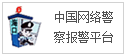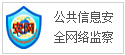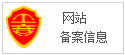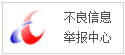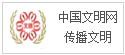# PyTorch系统性学习之入门介绍

pytorch 环境安装及配置

``# install pytorch 1.4!pip install -U torch torchvision -i https://mirrors.aliyun.com/pypi/simpleLooking in indexes: https://mirrors.aliyun.com/pypi/simpleCollecting torch  Using cached https://mirrors.aliyun.com/pypi/packages/1a/3b/fa92ece1e58a6a48ec598bab327f39d69808133e5b2fb33002ca754e381e/torch-1.4.0-cp37-cp37m-manylinux1_x86_64.whl (753.4 MB)Collecting torchvision  Using cached https://mirrors.aliyun.com/pypi/packages/1c/32/cb0e4c43cd717da50258887b088471568990b5a749784c465a8a1962e021/torchvision-0.5.0-cp37-cp37m-manylinux1_x86_64.whl (4.0 MB)Requirement already satisfied, skipping upgrade: six in /app/anaconda3/lib/python3.7/site-packages (from torchvision) (1.12.0)Requirement already satisfied, skipping upgrade: pillow>=4.1.1 in /app/anaconda3/lib/python3.7/site-packages (from torchvision) (6.2.0)Requirement already satisfied, skipping upgrade: numpy in /app/anaconda3/lib/python3.7/site-packages (from torchvision) (1.17.2)Installing collected packages: torch, torchvisionSuccessfully installed torch-1.4.0 torchvision-0.5.0# verificationfrom __future__ import print_functionimport torchx = torch.rand(5, 3)print(x)tensor([[0.3890, 0.3672, 0.2697],        [0.1633, 0.1091, 0.9061],        [0.0438, 0.5167, 0.5995],        [0.0546, 0.0019, 0.8384],        [0.5708, 0.0217, 0.3954]])# check gpu deviceimport torchtorch.cuda.is_available()True``

## Tensors

``from __future__ import print_functionimport torchx = torch.empty(5, 3)print(x)x = torch.rand(5, 3)print(x)x = torch.zeros(5, 3, dtype=torch.long)print(x)x = torch.tensor([5.5, 3])print(x)x = x.new_ones(5, 3, dtype=torch.double)      # new_* methods take in sizesprint(x)x = torch.randn_like(x, dtype=torch.float)    # override dtype!print(x)    print(x.size())tensor([[0., 0., 0.],        [0., 0., 0.],        [0., 0., 0.],        [0., 0., 0.],        [0., 0., 0.]])tensor([[0.5029, 0.7441, 0.5813],        [0.1014, 0.4897, 0.2367],        [0.2384, 0.6276, 0.0321],        [0.9223, 0.4334, 0.9809],        [0.1237, 0.3212, 0.0656]])tensor([[0, 0, 0],        [0, 0, 0],        [0, 0, 0],        [0, 0, 0],        [0, 0, 0]])tensor([5.5000, 3.0000])tensor([[1., 1., 1.],        [1., 1., 1.],        [1., 1., 1.],        [1., 1., 1.],        [1., 1., 1.]], dtype=torch.float64)tensor([[ 0.5468, -0.4615, -0.0450],        [ 0.5001, -0.9717, -0.6103],        [-0.5345,  0.1126, -0.0836],        [-0.5534,  0.5423, -1.1128],        [-1.3799,  1.3353, -1.6969]])torch.Size([5, 3])``

## Operaations

``y = torch.rand(5, 3)print(x+y)tensor([[ 1.0743, -0.4365,  0.7751],        [ 1.4214, -0.7803, -0.2535],        [ 0.3591,  0.7957,  0.0637],        [-0.3185,  0.5621, -0.9368],        [-0.7098,  1.5445, -1.5394]])print(torch.add(x, y))tensor([[ 1.0743, -0.4365,  0.7751],        [ 1.4214, -0.7803, -0.2535],        [ 0.3591,  0.7957,  0.0637],        [-0.3185,  0.5621, -0.9368],        [-0.7098,  1.5445, -1.5394]])result = torch.empty(5, 3)torch.add(x, y, out=result)print(result)tensor([[ 1.0743, -0.4365,  0.7751],        [ 1.4214, -0.7803, -0.2535],        [ 0.3591,  0.7957,  0.0637],        [-0.3185,  0.5621, -0.9368],        [-0.7098,  1.5445, -1.5394]])# Any operation that mutates a tensor in-place is post-fixed with an _. For example: x.copy_(y), x.t_(), will change x.y.add_(x)print(y)print(x[:, 1])tensor([[ 1.0743, -0.4365,  0.7751],        [ 1.4214, -0.7803, -0.2535],        [ 0.3591,  0.7957,  0.0637],        [-0.3185,  0.5621, -0.9368],        [-0.7098,  1.5445, -1.5394]])tensor([-0.4615, -0.9717,  0.1126,  0.5423,  1.3353])# Resizing: If you want to resize/reshape tensor, you can use torch.view:x = torch.randn(4, 4)y = x.view(16)z = x.view(-1, 8)  # the size -1 is inferred from other dimensionsprint(x.size(), y.size(), z.size())torch.Size([4, 4]) torch.Size() torch.Size([2, 8])# If you have a one element tensor, use .item() to get the value as a Python numberx = torch.randn(1)print(x)print(x.item())tensor([0.9993])0.999320387840271``

## Numpy Bridge

``# Converting a Torch Tensor to a NumPy Arraya = torch.ones(5)print(a)b = a.numpy()print(b)a.add_(1)print(a)print(b)tensor([1., 1., 1., 1., 1.])[1. 1. 1. 1. 1.]tensor([2., 2., 2., 2., 2.])[2. 2. 2. 2. 2.]# Converting NumPy Array to Torch Tensorimport numpy as npa = np.ones(5)b = torch.from_numpy(a)np.add(a, 1, out=a)print(a)print(b)[2. 2. 2. 2. 2.]tensor([2., 2., 2., 2., 2.], dtype=torch.float64)## CUDA Tensorsif torch.cuda.is_available():    device = torch.device("cuda")          # a CUDA device object    y = torch.ones_like(x, device=device)  # directly create a tensor on GPU    x = x.to(device)                       # or just use strings ``.to("cuda")``    z = x + y    print(z)    print(z.to("cpu", torch.double))       # ``.to`` can also change dtype together!tensor([1.9993], device='cuda:0')tensor([1.9993], dtype=torch.float64)``

Central to all neural networks in PyTorch is the autograd package. Let’s first briefly visit this, and we will then go to training our first neural network.The autograd package provides automatic differentiation for all operations on Tensors. It is a define-by-run framework, which means that your backprop is defined by how your code is run, and that every single iteration can be different.

## Tensor

torch.Tensor is the central class of the package. If you set its attribute .requires_grad as True, it starts to track all operations on it. When you finish your computation you can call .backward() and have all the gradients computed automatically. The gradient for this tensor will be accumulated into .grad attribute.

``x = torch.ones(2, 2, requires_grad=True)print(x)y = x + 2print(y)print(y.grad_fn)z = y * y * 3out = z.mean()print(z, out)tensor([[1., 1.],        [1., 1.]], requires_grad=True)tensor([[3., 3.],        [3., 3.]], grad_fn=)tensor([[27., 27.],        [27., 27.]], grad_fn=) tensor(27., grad_fn=)a = torch.randn(2, 2)a = ((a * 3) / (a - 1))print(a.requires_grad)a.requires_grad_(True)print(a.requires_grad)b = (a * a).sum()print(b.grad_fn)FalseTrue``

``out.backward()print(x.grad)tensor([[4.5000, 4.5000],        [4.5000, 4.5000]])x = torch.randn(3, requires_grad=True)y = x * 2while y.data.norm() < 1000:    y = y * 2print(y)tensor([-1138.8549,   484.4676,   417.7082], grad_fn=)v = torch.tensor([0.1, 1.0, 0.0001], dtype=torch.float)y.backward(v)print(x.grad)tensor([1.0240e+02, 1.0240e+03, 1.0240e-01])# stop autograd from tracking history on Tensors with .requires_grad=True either by wrapping the code block in with torch.no_grad():print(x.requires_grad)print((x ** 2).requires_grad)with torch.no_grad():    print((x ** 2).requires_grad)    # Or by using .detach() to get a new Tensor with the same content but that does not require gradients:print(x.requires_grad)y = x.detach()print(y.requires_grad)print(x.eq(y).all())TrueTrueFalseTrueFalsetensor(True)``

## Neural networks

A typical training procedure for a neural network is as follows:

• Define the neural network that has some learnable parameters (or weights)
• Iterate over a dataset of inputs
• Process input through the network
• Compute the loss (how far is the output from being correct)
• Propagate gradients back into the network’s parameters
• Update the weights of the network, typically using a simple update rule: weight = weight - learning_rate * gradient
``import torchimport torch.nn as nnimport torch.nn.functional as Fclass Net(nn.Module):    def __init__(self):        super(Net, self).__init__()        # 1 input image channel, 6 output channels, 3x3 square convolution        # kernel        self.conv1 = nn.Conv2d(1, 6, 3)        self.conv2 = nn.Conv2d(6, 16, 3)        # an affine operation: y = Wx + b        self.fc1 = nn.Linear(16 * 6 * 6, 120)  # 6*6 from image dimension        self.fc2 = nn.Linear(120, 84)        self.fc3 = nn.Linear(84, 10)    def forward(self, x):        # Max pooling over a (2, 2) window        x = F.max_pool2d(F.relu(self.conv1(x)), (2, 2))        # If the size is a square you can only specify a single number        x = F.max_pool2d(F.relu(self.conv2(x)), 2)        x = x.view(-1, self.num_flat_features(x))        x = F.relu(self.fc1(x))        x = F.relu(self.fc2(x))        x = self.fc3(x)        return x    def num_flat_features(self, x):        size = x.size()[1:]  # all dimensions except the batch dimension        num_features = 1        for s in size:            num_features *= s        return num_featuresnet = Net()print(net)Net(  (conv1): Conv2d(1, 6, kernel_size=(3, 3), stride=(1, 1))  (conv2): Conv2d(6, 16, kernel_size=(3, 3), stride=(1, 1))  (fc1): Linear(in_features=576, out_features=120, bias=True)  (fc2): Linear(in_features=120, out_features=84, bias=True)  (fc3): Linear(in_features=84, out_features=10, bias=True))params = list(net.parameters())print(len(params))print(params.size())  # conv1's .weight10torch.Size([6, 1, 3, 3])# feed a random inputinput = torch.randn(1, 1, 32, 32)out = net(input)print(out)tensor([[-0.0082, -0.0266,  0.0843,  0.0188,  0.1456, -0.1081, -0.0937,  0.0086,         -0.0356,  0.0723]], grad_fn=)# Zero the gradient buffers of all parameters and backprops with random gradients:net.zero_grad()out.backward(torch.randn(1, 10))``

## Loss Function

``output = net(input)target = torch.randn(10)  # a dummy target, for exampletarget = target.view(1, -1)  # make it the same shape as outputcriterion = nn.MSELoss()loss = criterion(output, target)print(loss)tensor(1.0206, grad_fn=)print(loss.grad_fn)  # MSELossprint(loss.grad_fn.next_functions)  # Linearprint(loss.grad_fn.next_functions.next_functions)  # ReLU``

## Backprop

``net.zero_grad()     # zeroes the gradient buffers of all parametersprint('conv1.bias.grad before backward')print(net.conv1.bias.grad)loss.backward()print('conv1.bias.grad after backward')print(net.conv1.bias.grad)conv1.bias.grad before backwardtensor([0., 0., 0., 0., 0., 0.])conv1.bias.grad after backwardtensor([-0.0241, -0.0161, -0.0086, -0.0032,  0.0125,  0.0005])``

## Update the weights

``# using sgdlearning_rate = 0.01for f in net.parameters():    f.data.sub_(f.grad.data * learning_rate)# using custom optimizer in torch.optimimport torch.optim as optim# create your optimizeroptimizer = optim.SGD(net.parameters(), lr=0.01)# in your training loop:optimizer.zero_grad()   # zero the gradient buffersoutput = net(input)loss = criterion(output, target)loss.backward()optimizer.step()    # Does the update``

## Training a classifier

When you have to deal with image, text, audio or video data, you can use standard python packages that load data into a numpy array. Then you can convert this array into a torch.*Tensor.

• For images, packages such as Pillow, OpenCV are usefu
• For audio, packages such as scipy and librosa
• For text, either raw Python or Cython based loading, or NLTK and SpaCy are useful

## Training an image classifier

• Load and normalizing the CIFAR10 training and test datasets using torchvision
• Define a Convolutional Neural Network
• Define a loss function
• Train the network on the training data
• Test the network on the test data

``import torchimport torchvisionimport torchvision.transforms as transformstransform = transforms.Compose(    [transforms.ToTensor(),     transforms.Normalize((0.5, 0.5, 0.5), (0.5, 0.5, 0.5))])trainset = torchvision.datasets.CIFAR10(root='./data', train=True,                                        download=True, transform=transform)trainloader = torch.utils.data.DataLoader(trainset, batch_size=4,                                          shuffle=True, num_workers=2)testset = torchvision.datasets.CIFAR10(root='./data', train=False,                                       download=True, transform=transform)testloader = torch.utils.data.DataLoader(testset, batch_size=4,                                         shuffle=False, num_workers=2)classes = ('plane', 'car', 'bird', 'cat',           'deer', 'dog', 'frog', 'horse', 'ship', 'truck')Files already downloaded and verifiedFiles already downloaded and verified%matplotlib inlineimport matplotlib.pyplot as pltimport numpy as np# functions to show an imagedef imshow(img):    img = img / 2 + 0.5     # unnormalize    npimg = img.numpy()    plt.imshow(np.transpose(npimg, (1, 2, 0)))    plt.show()# get some random training imagesdataiter = iter(trainloader)images, labels = dataiter.next()# show imagesimshow(torchvision.utils.make_grid(images))# print labelsprint(' '.join('%5s' % classes[labels[j]] for j in range(4)))````cat   cat  deer  ship``

### Define a Convolutional Neural Network

``import torch.nn.functional as Fimport torch.nn as nnclass Net(nn.Module):    def __init__(self):        super(Net, self).__init__()        self.conv1 = nn.Conv2d(3, 6, 5)        self.pool = nn.MaxPool2d(2, 2)        self.conv2 = nn.Conv2d(6, 16, 5)        self.fc1 = nn.Linear(16 * 5 * 5, 120)        self.fc2 = nn.Linear(120, 84)        self.fc3 = nn.Linear(84, 10)    def forward(self, x):        x = self.pool(F.relu(self.conv1(x)))        x = self.pool(F.relu(self.conv2(x)))        x = x.view(-1, 16 * 5 * 5)        x = F.relu(self.fc1(x))        x = F.relu(self.fc2(x))        x = self.fc3(x)        return x    net = Net()``

### Define a Loss function and optimizer

``import torch.optim as optimcriterion = nn.CrossEntropyLoss()optimizer = optim.SGD(net.parameters(), lr=0.001, momentum=0.9)``

### Train the network

``for epoch in range(2):  # loop over the dataset multiple times    running_loss = 0.0    for i, data in enumerate(trainloader):#         print(data)        # get the inputs; data is a list of [inputs, labels]        inputs, labels = data#         print(inputs, labels)        # zero the parameter gradients        optimizer.zero_grad()        # forward + backward + optimize        outputs = net(inputs)        loss = criterion(outputs, labels)        loss.backward()        optimizer.step()        # print statistics        running_loss += loss.item()        if i % 2000 == 1999:    # print every 2000 mini-batches            print('[%d, %5d] loss: %.3f' %                  (epoch + 1, i + 1, running_loss / 2000))            running_loss = 0.0print('Finished Training')[1,  2000] loss: 2.203[1,  4000] loss: 1.875[1,  6000] loss: 1.680[1,  8000] loss: 1.563[1, 10000] loss: 1.480[1, 12000] loss: 1.474[2,  2000] loss: 1.397[2,  4000] loss: 1.365[2,  6000] loss: 1.350[2,  8000] loss: 1.321[2, 10000] loss: 1.302[2, 12000] loss: 1.300Finished TrainingPATH = './cifar_net.pth'torch.save(net.state_dict(), PATH)dataiter = iter(testloader)images, labels = dataiter.next()# print imagesimshow(torchvision.utils.make_grid(images))print(labels)print('GroundTruth: ', ' '.join('%5s' % classes[labels[j]] for j in range(4)))````tensor([3, 8, 8, 0])GroundTruth:    cat  ship  ship planenet = Net()net.load_state_dict(torch.load(PATH))outputs = net(images)print(outputs)_, predicted = torch.max(outputs, 1)print(predicted)print('Predicted: ', ' '.join('%5s' % classes[predicted[j]]                              for j in range(4)))tensor([[-8.1609e-01, -7.3227e-01,  1.9178e-01,  1.9166e+00, -9.6811e-01,          8.3362e-01,  1.1127e+00, -1.4818e+00,  3.7150e-01, -7.1563e-01],        [ 7.2351e+00,  4.0154e+00,  1.7224e-03, -2.3247e+00, -2.0117e+00,         -4.3768e+00, -4.1172e+00, -4.6825e+00,  8.7625e+00,  2.0940e+00],        [ 3.9245e+00,  1.3894e+00,  6.4428e-01, -1.0531e+00, -8.4998e-01,         -2.2757e+00, -2.7469e+00, -2.2073e+00,  4.4428e+00,  6.6101e-01],        [ 4.5622e+00, -6.9576e-02,  1.1598e+00, -8.8092e-01,  9.0635e-01,         -2.1905e+00, -1.8022e+00, -2.2323e+00,  4.0340e+00, -7.8086e-01]],       grad_fn=)tensor([3, 8, 8, 0])Predicted:    cat  ship  ship planecorrect = 0total = 0with torch.no_grad():    for data in testloader:        images, labels = data        outputs = net(images)        _, predicted = torch.max(outputs.data, 1)        total += labels.size(0)        correct += (predicted == labels).sum().item()print('Accuracy of the network on the 10000 test images: %d %%' % (    100 * correct / total))Accuracy of the network on the 10000 test images: 55 %class_correct = list(0. for i in range(10))class_total = list(0. for i in range(10))with torch.no_grad():    for data in testloader:        images, labels = data        outputs = net(images)        _, predicted = torch.max(outputs, 1)        c = (predicted == labels).squeeze()        for i in range(4):            label = labels[i]            class_correct[label] += c[i].item()            class_total[label] += 1for i in range(10):    print('Accuracy of %5s : %2d %%' % (        classes[i], 100 * class_correct[i] / class_total[i]))Accuracy of plane : 66 %Accuracy of   car : 59 %Accuracy of  bird : 36 %Accuracy of   cat : 38 %Accuracy of  deer : 56 %Accuracy of   dog : 27 %Accuracy of  frog : 73 %Accuracy of horse : 59 %Accuracy of  ship : 72 %Accuracy of truck : 63 %``

### Training on GPU

``device = torch.device("cuda:0" if torch.cuda.is_available() else "cpu")# Assuming that we are on a CUDA machine, this should print a CUDA device:print(device)net.to(device)for epoch in range(2):  # loop over the dataset multiple times    running_loss = 0.0    for i, data in enumerate(trainloader):#         print(data)        # get the inputs; data is a list of [inputs, labels]        inputs, labels = data.to(device), data.to(device)#         print(inputs, labels)        # zero the parameter gradients        optimizer.zero_grad()        # forward + backward + optimize        outputs = net(inputs)        loss = criterion(outputs, labels)        loss.backward()        optimizer.step()        # print statistics        running_loss += loss.item()        if i % 2000 == 1999:    # print every 2000 mini-batches            print('[%d, %5d] loss: %.3f' %                  (epoch + 1, i + 1, running_loss / 2000))            running_loss = 0.0print('Finished Training')cuda:0[1,  2000] loss: 1.195[1,  4000] loss: 1.204[1,  6000] loss: 1.204[1,  8000] loss: 1.188[1, 10000] loss: 1.207[1, 12000] loss: 1.228[2,  2000] loss: 1.191[2,  4000] loss: 1.209[2,  6000] loss: 1.202[2,  8000] loss: 1.209[2, 10000] loss: 1.214[2, 12000] loss: 1.203Finished Trainingnet = Net()net.load_state_dict(torch.load(PATH))correct = 0total = 0with torch.no_grad():    for data in testloader:        images, labels = data        outputs = net(images)        _, predicted = torch.max(outputs.data, 1)        total += labels.size(0)        correct += (predicted == labels).sum().item()print('Accuracy of the network on the 10000 test images: %d %%' % (    100 * correct / total))Accuracy of the network on the 10000 test images: 55 %``

## Training on multi gpus

``if torch.cuda.device_count() > 1:  print("Let's use", torch.cuda.device_count(), "GPUs!")net = nn.DataParallel(net)net.to(device)for epoch in range(4):  # loop over the dataset multiple times    running_loss = 0.0    for i, data in enumerate(trainloader):#         print(data)        # get the inputs; data is a list of [inputs, labels]        inputs, labels = data.to(device), data.to(device)#         print(inputs, labels)        # zero the parameter gradients        optimizer.zero_grad()        # forward + backward + optimize        outputs = net(inputs)        loss = criterion(outputs, labels)        loss.backward()        optimizer.step()        # print statistics        running_loss += loss.item()        if i % 2000 == 1999:    # print every 2000 mini-batches            print('[%d, %5d] loss: %.3f' %                  (epoch + 1, i + 1, running_loss / 2000))            running_loss = 0.0print('Finished Training')Let's use 2 GPUs![1,  2000] loss: 1.202[1,  4000] loss: 1.197[1,  6000] loss: 1.202[1,  8000] loss: 1.194[1, 10000] loss: 1.211[1, 12000] loss: 1.210[2,  2000] loss: 1.208[2,  4000] loss: 1.184[2,  6000] loss: 1.215[2,  8000] loss: 1.198[2, 10000] loss: 1.201[2, 12000] loss: 1.208[3,  2000] loss: 1.206[3,  4000] loss: 1.209[3,  6000] loss: 1.198[3,  8000] loss: 1.206[3, 10000] loss: 1.209[3, 12000] loss: 1.208[4,  2000] loss: 1.203[4,  4000] loss: 1.206[4,  6000] loss: 1.203[4,  8000] loss: 1.187[4, 10000] loss: 1.220[4, 12000] loss: 1.207Finished Training``（正文已结束）

Copyright.2002-2019 格调中国网 版权所有 本网拒绝一切非法行为 欢迎监督举报 如有错误信息 欢迎纠正